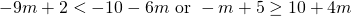Chapter 4: Inequalities

# 4.2 Compound Inequalities

Several inequalities can be combined together to form what are called compound inequalities.

The first type of compound inequality is the “or” inequality, which is true when either inequality results in a true statement. When graphing this type of inequality, one useful trick is to graph each individual inequality above the number line before moving them both down together onto the actual number line.

When giving interval notation for a solution, if there are two different parts to the graph, put a ∪ (union) symbol between two sets of interval notation, one for each part.

Example 4.2.1

Solve the inequality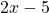>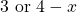>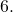Graph the solution and write it in interval notation.

Isolate the variables from the numbers: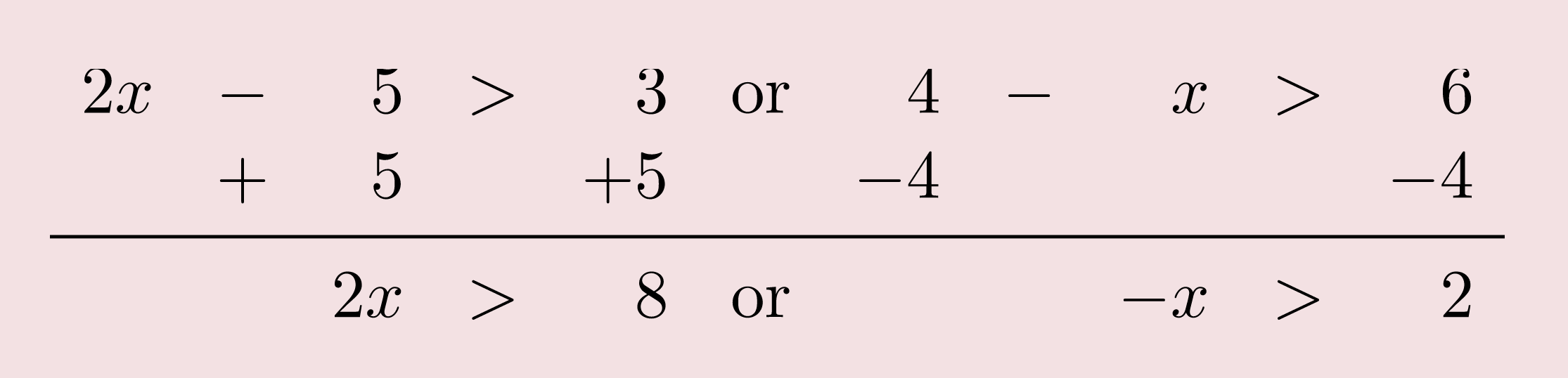Isolate the variable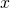(remember to flip the sense where necessary):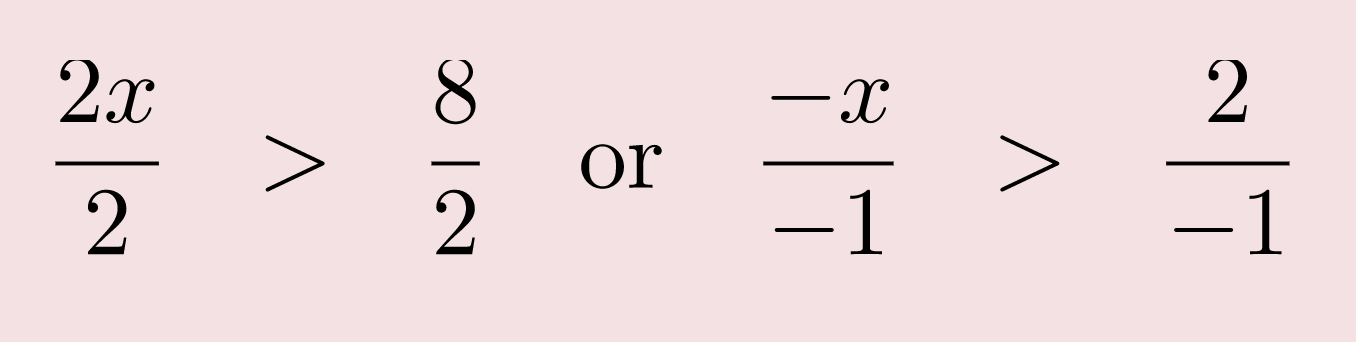Solution: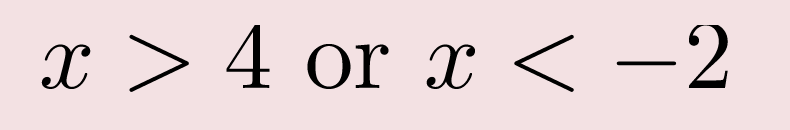Position the inequalities and graph: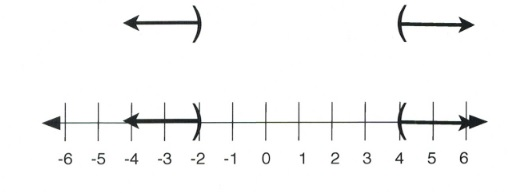In interval notation, the solution is written as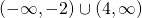.

Note: there are several possible results that result from an “or” statement. The graphs could be pointing different directions, as in the graph above, or pointing in the same direction, as in the graph representing>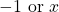>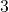that is shown below.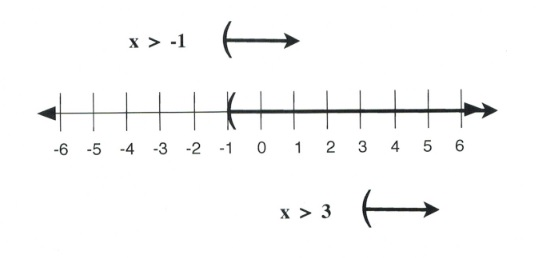In interval notation, this solution is written as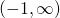.

It is also possible to have solutions that point in opposite directions but are overlapping, as shown by the solutions and graph below.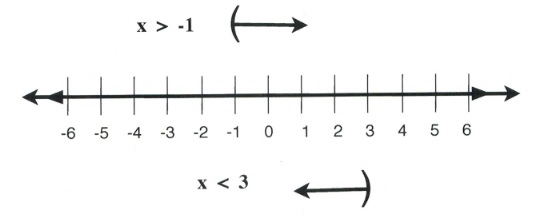In interval notation, this solution is written as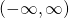, or simply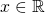,  since the graph is all possible numbers.

The second type of compound inequality is the “and” inequality. “And” inequalities require both inequality statements to be true. If one part is false, the whole inequality is false. When graphing these inequalities, follow a similar process as before, sketching both solutions for both inequalities above the number line. However, this time, it is only the overlapping portion that is drawn onto the number line. When a solution for an “and” compound inequality is given in interval notation, it will be expressed in a manner very similar to single inequalities. The symbol that can be used for “and” is the intersection symbol, ∩.

Example 4.2.2

Solve the compound inequality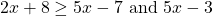>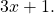Graph the solution and express it in interval notation.

Move all variables to the right side and all numbers to the left: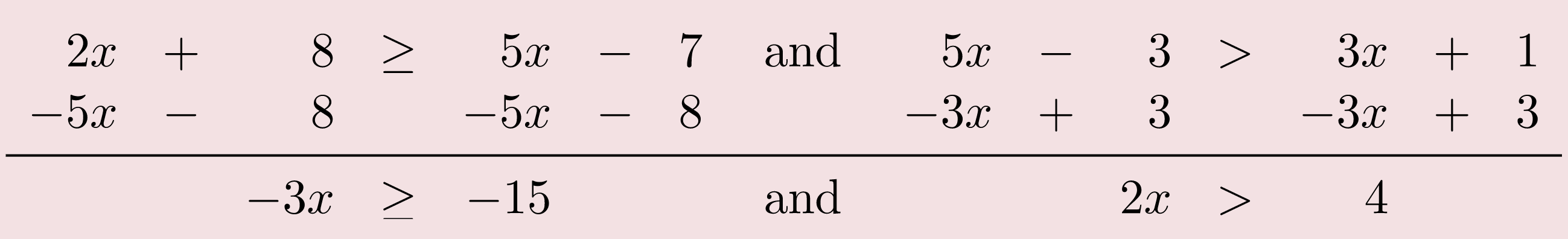Isolate the variablefor both (flip the sense for the negative):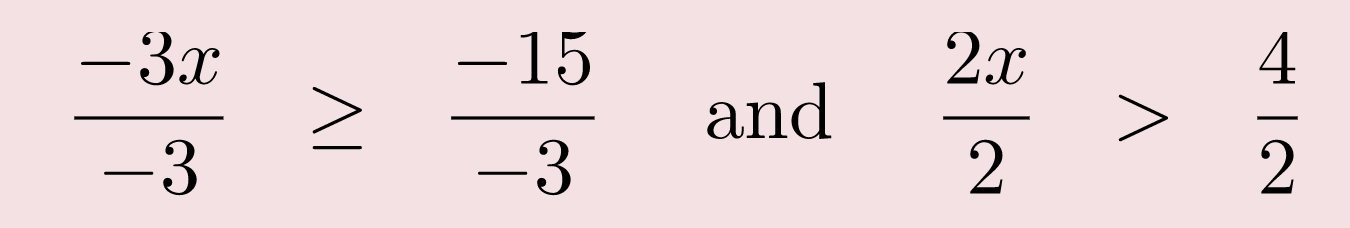Solution: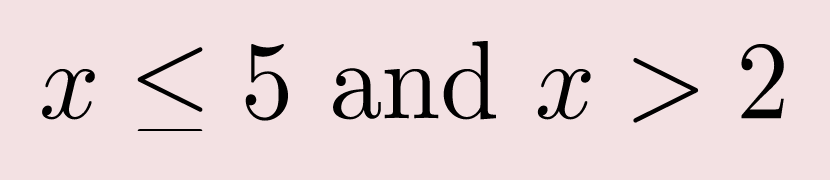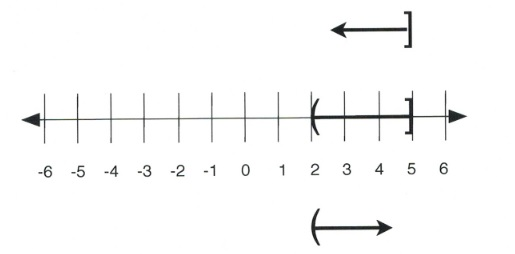In interval notation, this solution is written as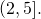Note: there are several different results that could result from an “and” statement. The graphs could be pointing towards each other as in the graph above, or pointing in the same direction, as in the graph representing>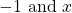>(shown below). In this case, the solution must be true for both inequalities, which make a combined graph of: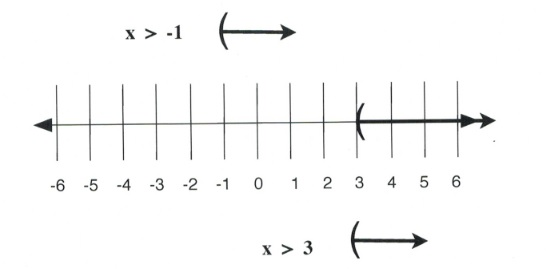In interval notation, this solution is written as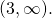It is also possible to have solutions that point in opposite directions but do not overlap, as shown by the solutions and graph below. Since there is no overlap, there is no real solution.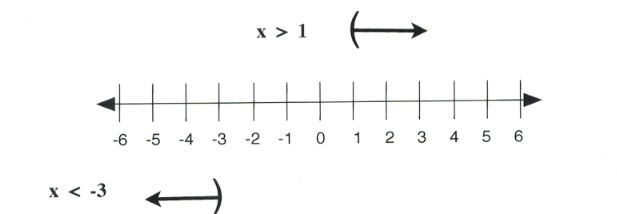In interval notation, this solution is written as no solution,or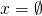.

The third type of compound inequality is a special type of “and” inequality. When the variable (or expression containing the variable) is between two numbers, write it as a single math sentence with three parts, such as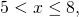to showis greater than 5 and less than or equal to 8. To stay balanced when solving this type of inequality, because there are three parts to work with, it is necessary to perform the same operation on all three parts. The graph, then, is of the values between the benchmark numbers with appropriate brackets on the ends.

Example 4.2.3

Solve the inequality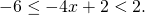Graph the solution and write it in interval notation.

Isolate the variable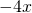by subtracting 2 from all three parts: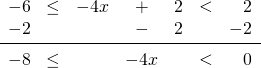Isolate the variableby dividing all three parts by −4 (remember to flip the sense):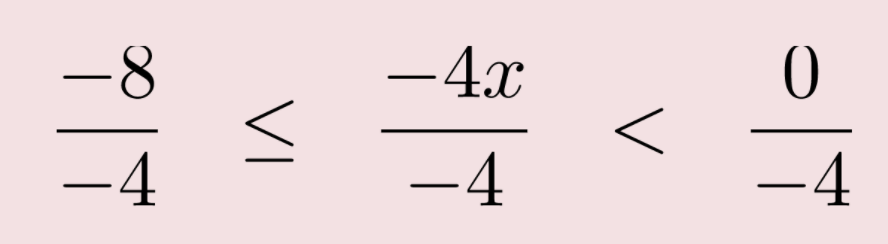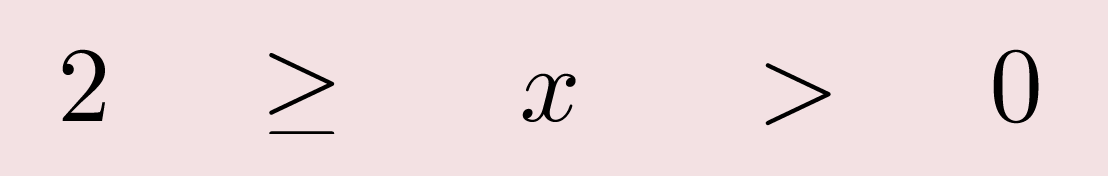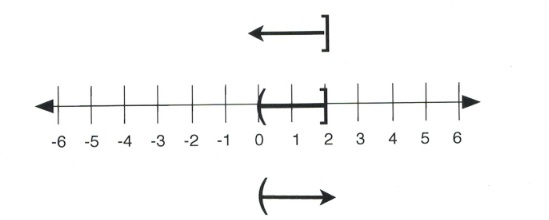In interval notation, this is written as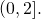# Questions

For questions 1 to 32, solve each compound inequality, graph its solution, and write it in interval notation.

1.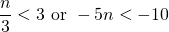2.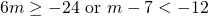3.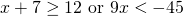4.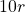>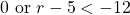5.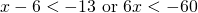6.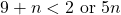>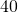7.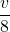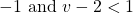8.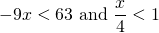9.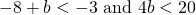10.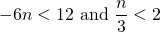11.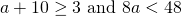12.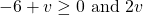>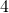13.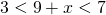14.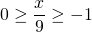15.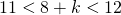16.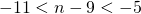17.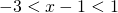18.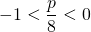19.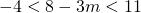20.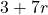>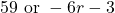>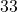21.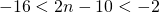22.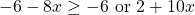>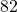23.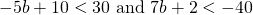24.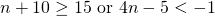25.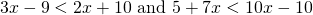26.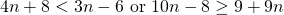27.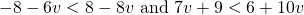28.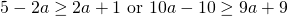29.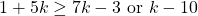>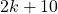30.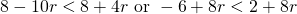31.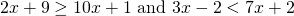32.